## 这个程序结果 我没看懂。。。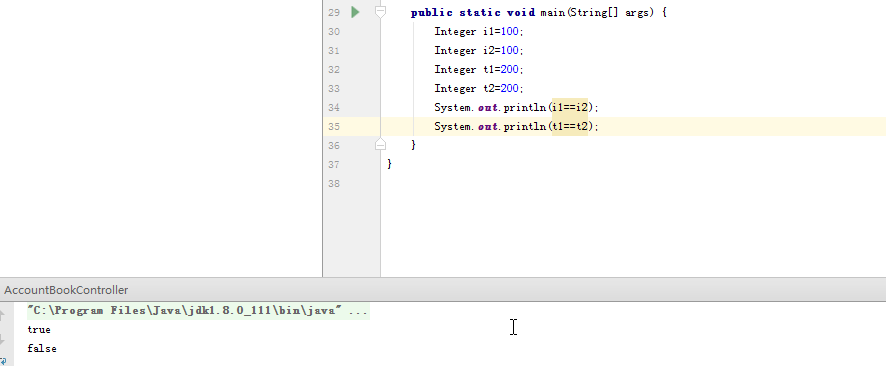1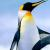IntegerCache   128 -127    自动装箱拆箱

``````public static Integer valueOf(int i) {
assert IntegerCache.high >= 127;
if (i >= IntegerCache.low && i <= IntegerCache.high)
return IntegerCache.cache[i + (-IntegerCache.low)];
return new Integer(i);
}  ``````

0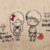java基础上有说过啊  超过199  就不能相等0
s

0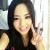#### 引用来自“蜗牛君”的评论

IntegerCache   128 -127    自动装箱拆箱

``````public static Integer valueOf(int i) {
assert IntegerCache.high >= 127;
if (i >= IntegerCache.low && i <= IntegerCache.high)
return IntegerCache.cache[i + (-IntegerCache.low)];
return new Integer(i);
}  ``````

0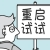==比较的是变量存的内存地址，  但是由于Integer的自动装箱机制，-128到127范围内的都是用同一个地址空间。

0
W
```/**
* Cache to support the object identity semantics of autoboxing for values between
* -128 and 127 (inclusive) as required by JLS.
*
* The cache is initialized on first usage.  The size of the cache
* may be controlled by the {@code -XX:AutoBoxCacheMax=<size>} option.
* During VM initialization, java.lang.Integer.IntegerCache.high property
* may be set and saved in the private system properties in the
* sun.misc.VM class.
*/

private static class IntegerCache {
static final int low = -128;
static final int high;
static final Integer cache[];

static {
// high value may be configured by property
int h = 127;
String integerCacheHighPropValue =
sun.misc.VM.getSavedProperty("java.lang.Integer.IntegerCache.high");
if (integerCacheHighPropValue != null) {
try {
int i = parseInt(integerCacheHighPropValue);
i = Math.max(i, 127);
// Maximum array size is Integer.MAX_VALUE
h = Math.min(i, Integer.MAX_VALUE - (-low) -1);
} catch( NumberFormatException nfe) {
// If the property cannot be parsed into an int, ignore it.
}
}
high = h;

cache = new Integer[(high - low) + 1];
int j = low;
for(int k = 0; k < cache.length; k++)
cache[k] = new Integer(j++);

// range [-128, 127] must be interned (JLS7 5.1.7)
assert IntegerCache.high >= 127;
}

private IntegerCache() {}
}```

```public static Integer valueOf(int i) {
if (i >= IntegerCache.low && i <= IntegerCache.high)
return IntegerCache.cache[i + (-IntegerCache.low)];
return new Integer(i);
}```

```Integer i1=127;
Integer i2=127;```

```Integer i3=128;
Integer i4=128;```

```Integer i5=new Integer(127);
Integer i6=new Integer(127);```

```Integer i1=127;
Integer i2=127;

System.out.println("i1是否和i2同地址 "+(i1==i2));

Integer i3=128;
Integer i4=128;

System.out.println("i3是否和i4同地址 "+(i3==i4));

Integer i5=new Integer(127);
Integer i6=new Integer(127);

System.out.println("i5是否和i6同地址 "+(i5==i6));```

i1是否和i2同地址 true
i3是否和i4同地址 false
i5是否和i6同地址 false

0

Integer 中有一个  byte[] 数组 用来缓存了 byte取值区间内的数字   自动装箱的时候 若数字是byte内的 则直接使用缓存中的